# Scikit-learn Pipelines: Custom Transformers and Pandas integration

Last updated:

See all examples on this Jupyter notebook

In addition to scoring, you can (and should) include all preprocessing steps into a single Pipeline:

• Selecting columns from Pandas dataframes

• Cleaning/preprocessing/normalizing data

• Imputting missing data

See more Scikit-learn Pipeline Examples

Why pipelines

• Make models easier to use, as it's a single object you can save/restore.

• Make models more reproducible because all preprocessing and modelling steps are done together.

• Reduce chance of data leaking because all operations are done separately on train and validation sets

• Make hyperparameter search easier becase it's a single object

Versions: Scikit-learn 0.22.2, Pandas 1.0.1

## Custom Dataframe Transformer

Create a custom Transformer that applies an arbitrary function to a pandas dataframe:

import pandas as pd
from sklearn.pipeline import Pipeline

class DataframeFunctionTransformer():
def __init__(self, func):
self.func = func

def transform(self, input_df, **transform_params):
return self.func(input_df)

def fit(self, X, y=None, **fit_params):
return self

# this function takes a dataframe as input and
# returns a modified version thereof
def process_dataframe(input_df):
input_df["text"] = input_df["text"].map(lambda t: t.upper())
return input_df

# sample dataframe
df = pd.DataFrame({
"id":[1,2,3,4],
"text":["foo","Bar","BAz","quux"]
})

# this pipeline has a single step
pipeline = Pipeline([
("lowercase", DataframeFunctionTransformer(process_dataframe))
])

# apply the pipeline to the input dataframe
pipeline.fit_transform(df)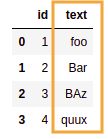BEFORE: source dataframe has a numeric
and one text column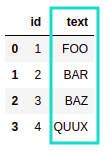AFTER: process_dataframe() function
applied to the source dataframe
via DataframeFunctionTransformer

## To Dense Transformer

Full code on this notebook

For example, you may need to add a step that turns a sparse matrix into a dense matrix, if you need to use a method that requires dense matrices such as GaussianNB or PCA:

from sklearn.base import TransformerMixin,BaseEstimator
from sklearn.decomposition import PCA
from sklearn.pipeline import Pipeline
from sklearn.tree import DecisionTreeClassifier

class ToDenseTransformer():

# here you define the operation it should perform
def transform(self, X, y=None, **fit_params):
return X.todense()

# just return self
def fit(self, X, y=None, **fit_params):
return self

# need to make matrices dense because PCA does not work with sparse vectors.
pipeline = Pipeline([
('to_dense',ToDenseTransformer()),
('pca',PCA()),
('clf',DecisionTreeClassifier())
])

pipeline.fit(sparse_data_matrix,target)
pipeline.predict(sparse_data_matrix)
# >>> array([1, 1, 1, 0, 0, 1, 1, 1])


## Select Columns Transformer

You can create a custom transformer that can go into scikit learn pipelines.

It just needs to implement fit and transform:

import pandas as pd

from sklearn.base import TransformerMixin,BaseEstimator
from sklearn.pipeline import Pipeline

class SelectColumnsTransformer():
def __init__(self, columns=None):
self.columns = columns

def transform(self, X, **transform_params):
cpy_df = X[self.columns].copy()
return cpy_df

def fit(self, X, y=None, **fit_params):
return self

df = pd.DataFrame({
'name':['alice','bob','charlie','david','edward'],
'age':[24,32,np.nan,38,20]
})

# create a pipeline with a single transformer
pipe = Pipeline([
('selector', SelectColumnsTransformer(["name"]))
])

pipe.fit_transform(df)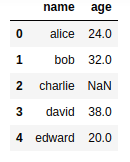BEFORE: Full dataframe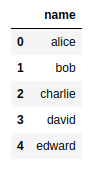AFTER: Only column name is selected

## ColumnTransformer: Missing imputation

Use ColumnTransformer passing a SimpleImputer:

import pandas as pd

from sklearn.compose import ColumnTransformer
from sklearn.pipeline import Pipeline
from sklearn.impute import SimpleImputer

df = pd.DataFrame({
'name':['alice','bob','charlie','david','edward'],
'age':[24,32,np.nan,38,20]
})

transformer_step = ColumnTransformer([
('impute_mean', SimpleImputer(strategy='mean'), ['age'])
], remainder='passthrough')

pipe = Pipeline([
('select', select)
])

# fit as you would a normal transformer
pipe.fit(features)

# transform the input
pipe.transform(features)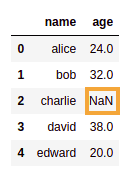BEFORE: NaN value in column 'age'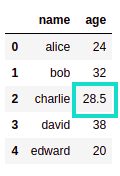AFTER: NaNvalue was replaced by
the mean of column 'age'
but the column 'name' was
not modified

## Pipeline with Preprocessing and Classifier

Create a single Pipeline that takes a DataFrame as input, does preprocessing (for all columns) using a ColumnTransformer and trains a DecisionTreeClassifier on top of it.

import numpy as np
import pandas as pd

from sklearn.compose import ColumnTransformer
from sklearn.impute import SimpleImputer
from sklearn.pipeline import Pipeline
from sklearn.preprocessing import OneHotEncoder
from sklearn.tree import DecisionTreeClassifier

# this is the input dataframe
df = pd.DataFrame({
'favorite_color':['blue','green','red','green','blue'],
'age': [10,15,10,np.nan,10],
'target':[1,0,1,0,1]
})

# define individual transformers in a pipeline
categorical_preprocessing = Pipeline([('ohe', OneHotEncoder())])
numerical_preprocessing = Pipeline([('imputation', SimpleImputer())])

# define which transformer applies to which columns
preprocess = ColumnTransformer([
('categorical_preprocessing', categorical_preprocessing, ['favorite_color']),
('numerical_preprocessing', numerical_preprocessing, ['age'])
])

# create the final pipeline with preprocessing steps and
# the final classifier step
pipeline = Pipeline([
('preprocess', preprocess),
('clf', DecisionTreeClassifier())
])

# now fit the pipeline using the whole dataframe
df_features = df[['favorite_color','age']]
df_target = df['target']

# call fit on the dataframes
pipeline.fit(df_features, df_target)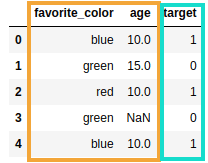DATAFRAME: This is the way most
people work like: a single dataframe
containing features (orange)
and the target (blue)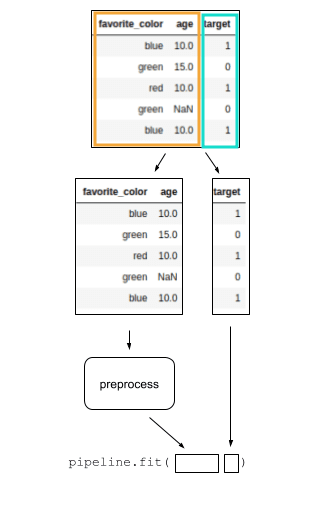PIPELINE FLOW: This is the
full pipeline, including
preprocessing and a classifier
at the end